Computational & Technology Resources an online resource for computational,engineering & technology publications not logged in - login Civil-Comp ProceedingsISSN 1759-3433 CCP: 80PROCEEDINGS OF THE FOURTH INTERNATIONAL CONFERENCE ON ENGINEERING COMPUTATIONAL TECHNOLOGY Edited by: B.H.V. Topping and C.A. Mota Soares Paper 31The Effect of Random Loads on Hydrodynamic Pressures A. Bayraktar and E. HançerDepartment of Civil Engineering, Karadeniz Technical University, Trabzon, Turkey doi:10.4203/ccp.80.31 Full Bibliographic Reference for this paper , "The Effect of Random Loads on Hydrodynamic Pressures", in B.H.V. Topping, C.A. Mota Soares, (Editors), "Proceedings of the Fourth International Conference on Engineering Computational Technology", Civil-Comp Press, Stirlingshire, UK, Paper 31, 2004. doi:10.4203/ccp.80.31 Keywords: random loads, hydrodynamic pressures, Lagrangian approach. Summary Dam-reservoir systems are fluid-structure interaction problems. Most fluid-structure interaction analyses are based on simplifying assumptions (e.g. inviscid flow) which allow one of two approaches: (1) displacements are the variables in the solid, pressures (or velocity potentials) are the variables in fluid (Eulerian approach); (2) displacements are the variables in both the fluid and solid (Lagrangian approach). In the Lagrangian approach, compatibility and equilibrium are automatically satisfied at the nodes along the interfaces between the fluid and structure. This makes a Lagrangian displacement-based fluid finite element very desirable, which can be readily incorporated into a general-purpose computer program for structural analysis, because special interface equations are not required. The aim of the present paper is to determine the effect of random loads on hydrodynamic pressures occurring on the upstream face of the dams by using the Lagrangian approach. For that reason, variable-number-nodes two-dimensional fluid finite elements proposed by Wilson and Khalvati  were programmed in Fortran language by the authors and incorporated into a general-purpose computer program for stochastic dynamic analysis of structure systems, STOCAL . For application, Sariyar concrete gravity dam, constructed in Turkey, is selected. Dam-reservoir interaction system is modelled by finite element method. There are two unknown displacements at each nodal point of the dam and reservoir. The dam and the reservoir are represented by 15 eight-noded and 24 nine-noded isoparametric quadrilateral solid and fluid finite elements, respectively. The fluid is assumed to be inviscid in this study. The normal components of the displacements of the reservoir-dam interfaces are to be continuous. This condition is accomplished by using short and axially almost rigid truss elements in the normal direction of the interfaces. The reservoir height is 85 m and the length in the upstream direction is taken to be as much as three times the reservoir height. It is assumed that the reservoir has constant depth. Element matrices are computed using 2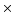2 and 33 integration orders for fluid and solid systems, respectively . Plane strain conditions are taken into account in the calculations. The dam material is assumed to be linear-elastic, homogeneous and isotropic. For the dam, the elasticity modulus, the unit weight and Poisson's ratio are chosen as 3510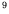N/m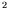, 2410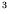N/m, 0.20, respectively. For the reservoir, the bulk modulus and the mass density are taken as 20710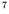N/m, 1000 kg/m, respectively. The value of the rotational constraint parameter is taken as 100 times of the bulk modulus . The first 20 modes are taken into account in this study . A stationary assumption is made for the stochastic dynamic analysis where the statistical parameters are independent of time. The Pacoima Dam record S16E component recorded during the San Fernando earthquake in 1971 is selected as a ground motion and applied to the dam in horizontal and vertical direction, separately. For vertical direction, two thirds of the earthquake motion is taken into account. The power spectral density function of S16E component of San Fernando earthquake is determined with the Fourier transforms of the autocorrelation function. Hydrodynamic pressures occurring on the upstream face of the dam under random excitation are calculated and compared with the absolute maximum hydrodynamic pressures obtained from deterministic dynamic analysis. Hydrodynamic pressures are obtained using the time interval of 0.005 second. A damping value of 5% is chosen for all modes of the coupled system. Deterministic and random hydrodynamic pressure along the upstream face of the dam are calculated at Gauss points which are in the fluid elements near the reservoir-dam interface for horizontal and vertical ground motion. Random hydrodynamic pressures are smaller than those of the deterministic for both cases of the ground motion. Since the mean of maximum values are obtained by averaging all the maximum response values in stochastic dynamic analysis, it should be expected that the absolute maximum values determined from deterministic dynamic analysis will be greater than the mean of maximum values obtained from stochastic dynamic analysis. References 1 Wilson, E.L., Khalvati, M., "Finite Elements for the Dynamic Analysis of Fluid-Solid systems", International Journal for Numerical Methods in Engineering, 19, 1657-1668, 1983. doi:10.1002/nme.1620191105 2 Button, M.R., Der Kiureghian, A., Wilson, E.L., "STOCAL-User Information Manual", Report No. UCB-SESM/81-2, Department of Civil Engineering, University of California, Berkeley, CA, 1981. 3 Bathe, K-J., "Finite Element Procedures in Engineering Analysis" New Jersey: Prentice-Hall Inc. Englewood Cliffs, 1982. 4 Calayir, Y., Dumanoglu, A.A., "Static and Dynamic Analysis of Fluid and Fluid-Structure Systems by the Lagrangian Method", Computers and Structures, 49, (4), 625-632, 1993. doi:10.1016/0045-7949(93)90067-N purchase the full-text of this paper (price £20) Back to top ©Civil-Comp Limited 2023 - terms & conditions